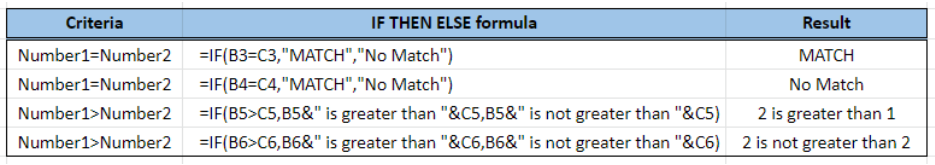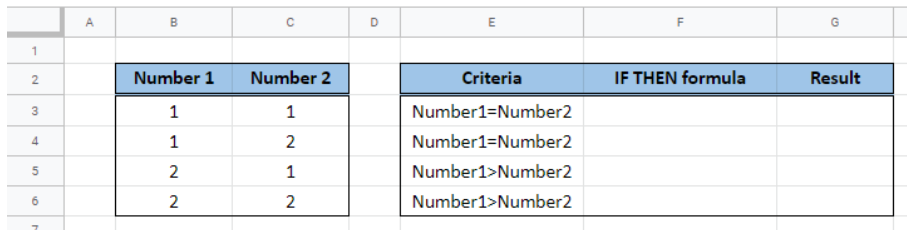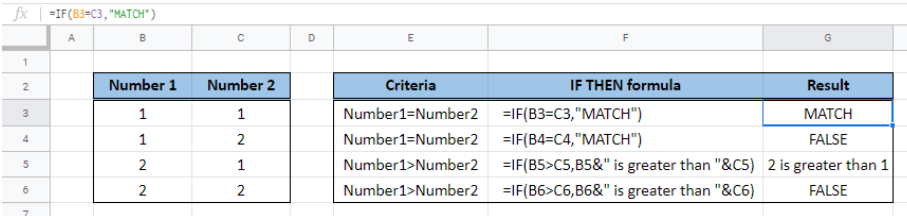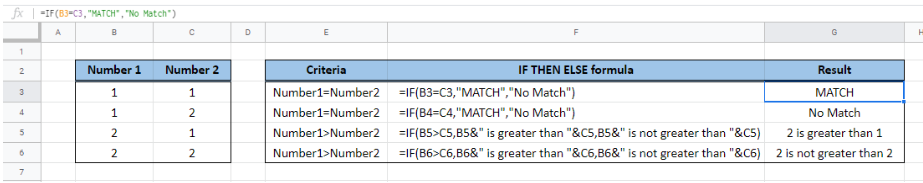Get instant live expert help with Excel or Google Sheets“My Excelchat expert helped me in less than 20 minutes, saving me what would have been 5 hours of work!”

#### Post your problem and you’ll get expert help in seconds.

Your message must be at least 40 characters
Our professional experts are available now. Your privacy is guaranteed.
All articles if then Google Sheets IF function

# Google Sheets IF function

IF function is one of the most widely used functions in Excel.  It evaluates a given logical test and returns either a TRUE or a FALSE.  In Google sheets, the syntax and usage of IF function is the same as in Excel.

While working with Google sheets, we are able to evaluate a criteria and set a corresponding action if TRUE, or another action if FALSE.Figure 1.  Final result:  IF then statements in Google sheets

## Syntax of IF function

An IF statement in Google sheets has the following syntax:

`=IF(logical_test, [value_if_true], [value_if_false])  `

• The arguments “value_if_true” and “value_if_false” are optional.  If left blank, the function will return TRUE if the logical test is met, and FALSE if otherwise.

## Google sheets IF Then

IF Then statements in Google sheets consists of a condition that needs to be evaluated and a corresponding action if the condition is met.  If the condition is not met, the function simply returns “FALSE”.

Suppose we have below list of Numbers in column B and column C.  For the first two sets of values, we want to evaluate if the first number is equal to the second number.  For the last two sets of values, we want to evaluate if the first number is greater than the second number.Figure 2.  Sample data for Google sheets IF then

Enter the following Google sheets IF then formulas in cells G3, G4, G5 and G6:

In G3: `=IF(B3=C3,"MATCH")`

In G4: `=IF(B4=C4,"MATCH")`

In G5: `=IF(B5>C5,B5&" is greater than "&C5)`

In G6: `=IF(B6>C6,B6&" is greater than "&C6)`

The Google sheets IF statements above evaluate the specified condition, then return the specified action if the condition is TRUE.  Otherwise, the IF function returns “FALSE”, since there is no action specified in the formula once the condition is not met.

The resulting value in G3 is the word “MATCH”, since B3 “1” is equal to C3 “1”.  On the other hand, the result in G4 is “FALSE”, since B4 “1” is not equal to C4 “2”.

In cell G5, the result is the THEN statement “2 is greater than 1” after the IF function evaluates the condition “B5>C5” to be TRUE.  In cell G6, however, the result is FALSE since “2” in B6 is not greater than “2” in C6.Figure 3.  Output: Google sheets IF then

## Google sheets IF Then Else

Google sheets IF Else statements consist of a condition that needs to be evaluated, a corresponding action if the condition is met, and another specified action if the condition is not met.

For the same list of numbers as in previous example, we want to:

• Return “MATCH” if the first two sets of values are evaluated as equal; Otherwise, indicate “No Match
• Determine if the first number is greater than the second number;  Otherwise, indicate that the first number is not greater than the second number

Enter the following formula in cells G3, G4, G5 and G6:

In G3: `=IF(B3=C3,"MATCH","No Match")`

In G4: `=IF(B4=C4,"MATCH","No Match")`

In G5: `=IF(B5>C5,B5&" is greater than "&C5,B5&" is not greater than "&C5)`

In G6: `=IF(B6>C6,B6&" is greater than "&C6,B6&" is not greater than "&C6)`

The difference between IF Then statements in Google sheets and IF Then Else statements is that in IF Then, the formula returns the word “FALSE” when a criteria is not met.  While in IF Then Else, the formula returns a user-specified action if the criteria is not met.Figure 4.  Output: Google sheets IF then else

### Leave a Comment## Subscribe to Excelchat.co

Get updates on helpful Excel topicsTrusted by people who work at